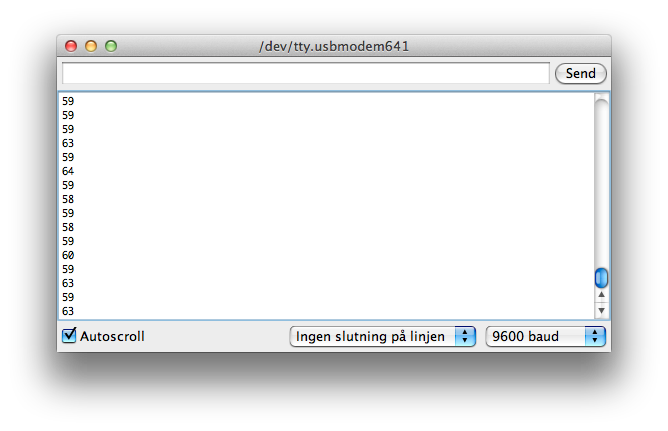# Help with frequency counter

Hello everyone,

I'm doing a school project with a few friends, and we're not very experienced with Arduino programming. By using the library from this website http://www.avdweb.nl/arduino/hardware-interfacing/frequency-period-counter.html#h0-1-8-multiple-frequency-inputs we're trying to measure a frequency of about 60 Hz. That is currently working without any problems (see the attached image).

Since we're using infrared to transmit the frequency noise can often be a problem. We basically want to compare the last 2 or 3 digits, so we know for sure the frequency is the right one. How can we do this in a simple way?

Thanks!Which version do I take:

Since we're using infrared to transmit, the frequency noise can often be a problem.

Since we're using infrared to transmit the frequency, noise can often be a problem.

If it is the former, you may need to stabilize the signal using low/high pass filters. However, the latter may indicate the noise is on the infra-red side, possibly from the sun, maybe sending some CRC/recovery bytes to verify the data will help.
If neither, produce the code...

A few more details would be helpful. How fast do you want to sample the frequency? Every cycle, couple of cycles, second, minute, hour? What exactly are you transmitting via IR, the signal that you are counting the frequency on, or your frequency count? Oh, and you should probably post your current code withing code blocks (use the # button in the message toolbar).

One method that you might consider is taking several samples and finding the mean. This is different than taking an average. As you know, with an average you find the mathematical middle point of your samples. With a mean, you sort your samples and pick the middle one. With the mean any outliers (i.e. noise) can't effect the actual value. I believe there is a library out there that should do this for you as discussed in another thread here. I'll try to find it at some point today if you don't beat me to it.

Sembazuru:
One method that you might consider is taking several samples and finding the mean. This is different than taking an average.

Unless otherwise specified, "average" is usually understood to be the arithmetic mean.

With a mean, you sort your samples and pick the middle one.

That'd be the median.

Right... Sorry for the term confusion.
I.E. what he said.pYro_65:

Since we're using infrared to transmit the frequency, noise can often be a problem.

That's what I meant, sorry! We were just testing the simple example on the website http://www.avdweb.nl/arduino/hardware-interfacing/frequency-period-counter.html, which uses the following code:

``````#include <FreqPeriodCounter.h>

const byte counterPin = 3;
const byte counterInterrupt = 1; // = pin 3
FreqPeriodCounter counter(counterPin, micros, 0);

void setup(void)
{ Serial.begin(9600);
attachInterrupt(counterInterrupt, counterISR, CHANGE);
}

void loop(void)
}

void counterISR()
{ counter.poll();
}
``````

I was wondering whether this "Smoothing" thing http://arduino.cc/en/Tutorial/Smoothing would do the trick. As far as I understand, it'll make an array of different incoming values and calculate the average number.

That smoothing example would work. However, using an array to store all those values is overkill if you only want the average. The average can be calculated with two variables--the sum and the count. No need to store each of the incoming values. The easiest way I can think of is to use a hard coded count. For example:

``````int Count;
int Total;

Total = 0;
for (Count = 0; Count < 100; ++Count)
Total += counter.hertz;

Serial.println(Total / 100.0);
``````

Unless you want to do something fancy with the outliers. For example, read 10 incoming values, toss out the highest two and the lowest two and average the rest. This should give you slightly more consistent results, but usually isn't necessary.